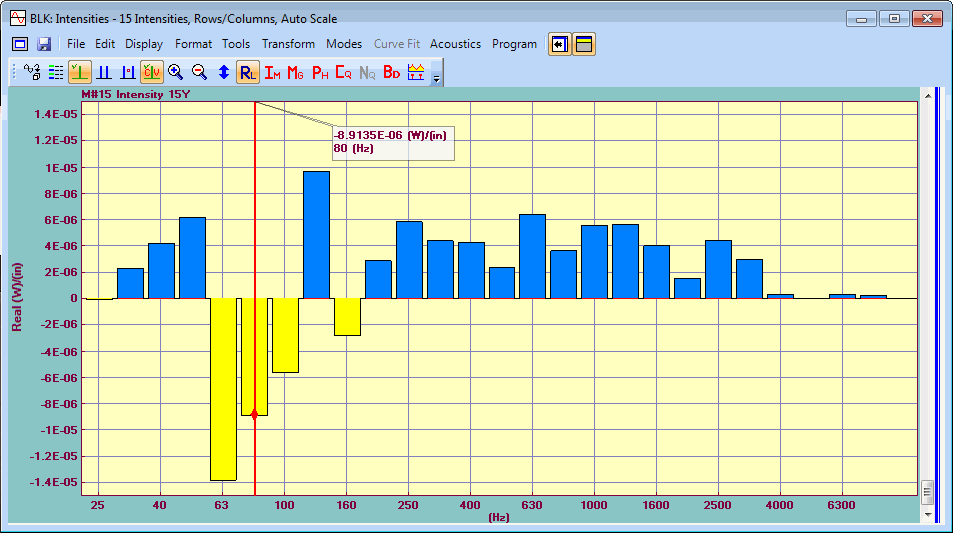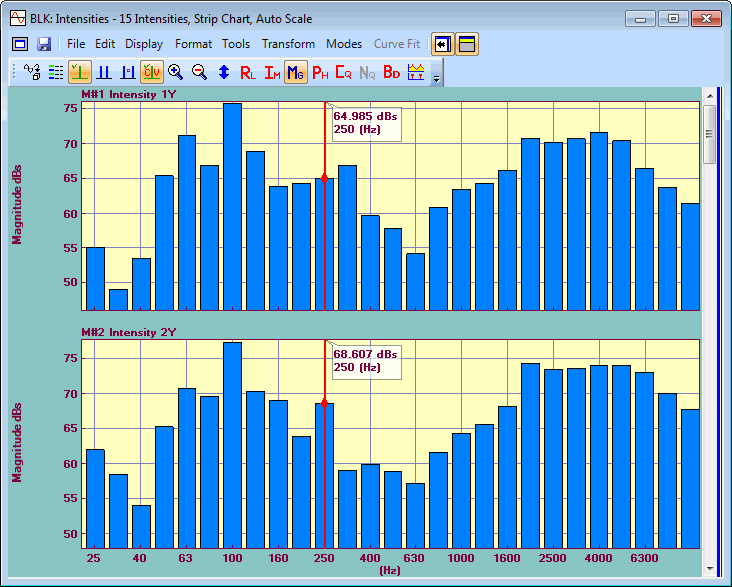## Display | Real

Displays either real measurement data, or the Real part of complex data in Octave band or dB Reference units.Data Block Window Showing Real Part of Octave Data.

## Octave Band Data

Log or dB formats can be chosen for displaying the Real part of Octave band data.  Linear, Log, dB & decades choices are made in the Format | Y-Axis dialog box.

• Right click in the M# graphics area, and execute Format | Y-Axis from the menu to open the Y-Axis dialog box.

## Real Part in dB Reference Units

The Real part can also be displayed in dB (decibel) units relative to a reference level. (See Display | Magnitude for details.)  For Linear (RMS) data, the Real part is displayed as,

Real part (dB Reference) = Sign (Real part) [ 20 Log10( Abs (Real part) / Linear Reference) ]

For Power (MS) data, the Real part is displayed as,

Real part (dB Reference) = Sign (Real part) [ 10 Log10( Abs (Real part) / Power Reference) ]

## Display | Magnitude

Displays the magnitude of the measurement data.Data Block Window Showing Log Magnitude of Octave Data.

## Linear, Log or dB

Magnitudes can be displayed in Linear, Log or dB format.  When Log or dB is chosen, you can also choose 1 to 14 decades (powers of 10) to display.

• Linear, Log, dB & decades choices are made in the Format | Y-Axis dialog box.

• Right click in the M# graphics area, and execute Format | Y-Axis from the menu to open the Y-Axis dialog box.

## dB Units for Linear Versus Power Quantities

Magnitudes can be displayed in dB (decibel) units.  For Linear (RMS) quantities, (such as FRFs, Linear Spectra, etc.) the Magnitude is displayed as,

Magnitude (dB) = 20 Log 10( Magnitude )

For Power (MS) quantities, (such as Auto Power Spectra, PSD’s, etc.), the Magnitude is displayed as,

Magnitude (dB) = 10 Log 10( Magnitude )

## Magnitude in dB Reference Units

Magnitudes can also be displayed in dB (decibel) units relative to a reference level.  For Linear (RMS) quantities, (such as SPL ) the Magnitude is displayed as,

Magnitude (dB Reference) = 20 Log 10( Magnitude / Linear Reference)

For Power (MS) quantities, (such as Sound Power & Intensity), the Magnitude is displayed as,

Magnitude (dB Reference) = 10 Log 10( Magnitude / Power Reference)

To display magnitudes in dB Reference units, the Linear (RMS) Reference or Power (MS) Reference values must be entered in the dB References column in the M#s spreadsheet.

The Linear (RMS) Reference value is required for Linear (RMS) M# data
The Power (MS) Reference value is required for Power (MS) M# data.